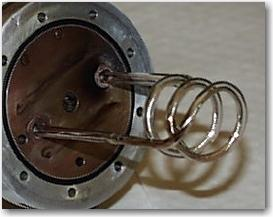# The procedure to do the impedance measurement

Status
Not open for further replies.

#### blueblin

##### Banned
Impedance Measurement

Hi All,

I would like to know the procedure to do the impedance measurement. I have a set of dipole antenna at 915MHz. I know it needs S11 to do the testing and calculation. Can anyone provide me the details for the measurement? Thank you very much..

Re: Impedance Measurement

Hi

well you need Vector network analyser (VNA) to measure your dipole S11 and impedance

i hope it helps

regards

Re: Impedance Measurement

If you don’t have a Network Analyzer you can measure the VSWR (and Return Loss) using a signal generator, a directive coupler, a detector (or a spectrum analyzer), and a matched load.
Γ=(VSWR-1)/(VSWR+1)
RL=-20*LOG(Γ)
In this way you measure only the real part of the impedance.

How can I measure the impedance of a small coil like the attached photo? Coil is 2mm coper wire located inside a quartz tube (4 mm diameter for water cooling). Coil diameter is 2.5 cm and lenght is 2.8 cm both like this and while plasma is ignited and coil is operating?The coil impedance will have two values, one when low power hits it, and an entirely different impedance when the plasma is initiated.

Obviously, this is a high power application, so you have to couple off a small amount of power to do the measurement. You do not mention the frequency, which is important. But in general you use two directional couplers, one measuring the power heading toward the coil, and one measuring the power reflected from the coil. Then you make the actual measurement on two much smaller samples of power to and away from the coil. (This assumes that you are connecting the coil to the RF power source using a controlled impedance transmission line.)

Once you have the two sampled powers, you can simply measure their power levels in watts to determine the return loss (or VSWR). For this you only need a power meter. If you want the actual impedance (real and reactive part), you will need to use a network analyzer, of it low enough frequency you could use a 2 channel oscilloscope and look at the amplitude and phase differences.

Rich
www.MaguffinMicrowave.com

The information is another thread. The discussion should be better continued there, I think.

Status
Not open for further replies.